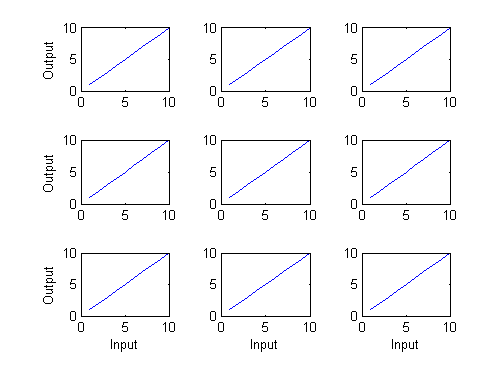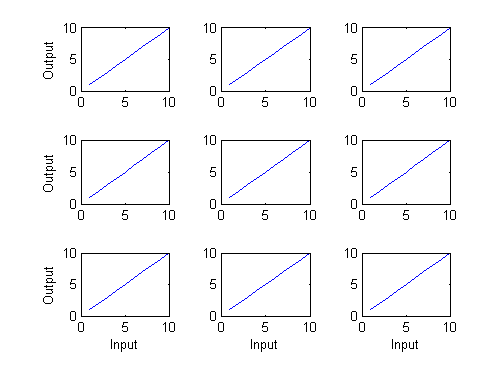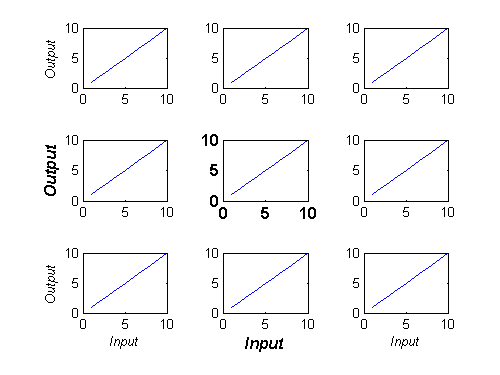# labelEdgeSubPlots

Bob's pick this week is labelEdgeSubPlots by Rob Campbell.

• Do you use subplot a lot in your MATLAB analyses?
• Do your subplots share the same X and Y range?
• Do you like to label axes only along the left and bottom?

You could write code to do that yourself. It's not difficult.

for k=1:9
ax(k) = subplot(3,3,k);
plot(1:10)
if mod(k,3)==1 %left
ylabel Output
end
if k>6 %bottom
xlabel Input
end
endOr you could just use labelEdgeSubPlots.

for k=1:9
ax(k) = subplot(3,3,k);
plot(1:10)
end
h = labelEdgeSubPlots('Input','Output')
h =
xlabels: [220.01 216.01 212.01]
ylabels: [213.01 201.01 187.01]Notice it returns axes handles. That helps customize your plots as much as you wish.

%make all axes labels italic
set([h.ylabels h.xlabels],'FontAngle','italic')

%draw special attention to center plot (12pt bold)
set([ax(5) h.xlabels(2) h.ylabels(2)],'FontWeight','bold','FontSize',12)Another nifty feature involves how labelEdgeSubPlots handles sparse subplots as shown in Rob's example screen shot.